#### You may also like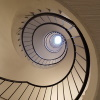### Tiny Nines

What do you notice about these families of recurring decimals?

# Repetitiously

##### Age 14 to 16Challenge Level

Arth from Tanglin Trust School in Singapore considered the case $x = 2.\dot2$:

If $x=0.\dot2$ and we have to get to $2.\dot2$

Then $x+2 = 2.\dot2$

Sanika P from PSBBMS in India continued:

$10x = 2.\dot2$
Therefore,
\begin{align} x+2&=10x\\ 9x&=2\\ x&=\tfrac29\end{align}

Josh from Winchcombe School in the UK, Mahdi from Mahatma Gandhi International School in India and Sanika P also expressed $0.\dot2\dot5$ as a fraction. This is Sanika's work:

$y = 0.2525...$
$y+25 = 25.2525...$
$y\times100 = 25.2525...$

Therefore,
\begin{align} y\times100&=y+25\\ 99y&=25\\ y&=\tfrac{25}{99}\end{align}

Jacob from Acland Burghley in the UK, Dylan from Thomas Starr King Middle School in the USA and Sanika P all described a general method for writing decimals like this as fractions. Dylan wrote:

Let's call this decimal $x.$ If all of $x$ repeats, like $0.\dot1\dot5,$ then multiply $x$ by a multiple [power] of $10$ so that $1$ repetition of $x$ is on the left of the decimal point. Now subtract $x$ from that value and now $x$ should have a coefficient equal to a whole number. Divide the whole number by the coefficient of $x$ and you have a fraction.

Jacob wrote a method which almost jumps straight to the answer:

1. Multiply $n$ by $10^\text{the number of recurring digits}$
2. Subtract $n$ to get $n \times (10^\text{the number or recurring digits} - 1)$
3. Divide by $10^\text{the number or recurring digits} - 1$
4. Done

And Dylan added a short cut to jump straight to the answer:

This all can be shortcut by taking one repetition and putting it over a number made of $9$s with the same [number] of digits, I.e. $0.\dot3\dot2$ equals $\frac{32}{99}.$ Of course if you need to explain how you got your answer then the method above shows each step.
See the problem Tiny Nines for more on this idea

Josh wrote this using sigma notation. Click to see Josh's work.

A recurring decimal $z$ that has $n$ alternating digits and can be represented in the form$$z=\sum_{i=0}^{\infty}{\frac{1}{{10}^{in}}\left(\sum_{j=1}^{n}\frac{a_j}{{10}^j}\right)}$$ where $a_j$ are integers between $1$ and $9$ inclusive that represent the [recurring] digits.

Then $$z=\frac{{10}^n}{{10}^n-1}\sum_{j=1}^{n}\frac{a_j}{{10}^j}=\frac{1}{{10}^n-1}\sum_{j=1}^{n}{{10}^{n-j}a_j}$$

Sanika P and Mahdi also expressed the other recurring decimals as fractions. This is Mahdi's work: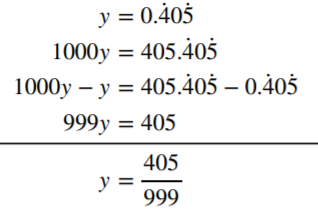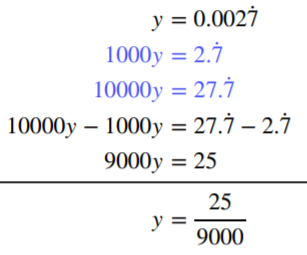Luke, Dylan and Sanika P all described a general method for recuring decimals like these. This is Sanika's method:

Let us take the decimal $0.23444...$ to elaborate.
Let $x$ be equal to $0.2344...$
We first multiply $x$ by $100$ to get a fully recurrent decimal part.
$x\times100= 23.44...$
We then multiply $x$ by $1000$ so that exactly one 1 iteration comes to the whole number part: $x\times1000=234.444...$
Now, we have obtained the same decimal part for both the case which makes it easier for us to subtract [one equation from the other] to get the following:
$x\times900=211$
Therefore, $x= \frac{211}{900}.$

Sanika also used a slightly different method for one of the examples:

$y = 0.8333...$
\begin{align}8.333... &= y\times10\\ 8.333 &=y+7.5\end{align}

Therefore,
\begin{align}y\times10&=y+7.5\\ 9y&=7.5 \\ y&=\tfrac{75}{90}\end{align}

Dylan described this method:

If not all of the decimal repeats, like $0.8\dot3,$ then the process is slightly different. Let us still call this decimal $x.$ First you need to multiply $x$ by a multiple [power] of $10$ so that the part that does not repeat is on the left of the decimal point. Now subtract $x$ from this value. You should get a number that has a few decimal points but does terminate. Let us call this value $y.$
(Note that you might need to multiply by a different power of $10$ to make sure that the recurring digits line up)

Now set up an equation with the $x$ multiplied by the [power of $10$] on one side and $x+y$ on the other. Solve this equation to find $x$ as a fraction. You may need to multiply the whole equation by a value equal to $1$ to remove any decimals from the fraction, or simplify.

Mahdi generalised Sanika's method algebraically. Click to see Mahdi's work.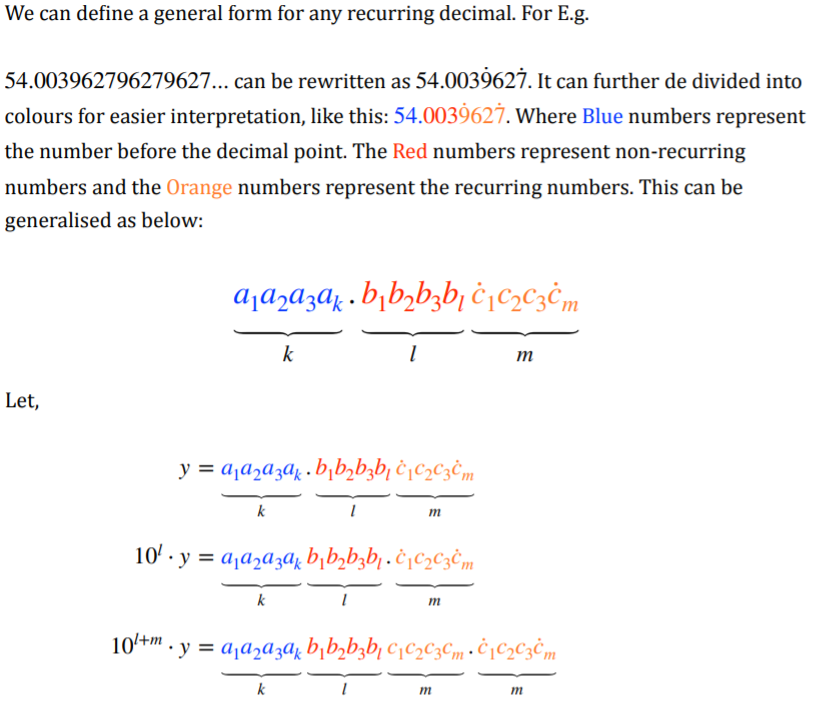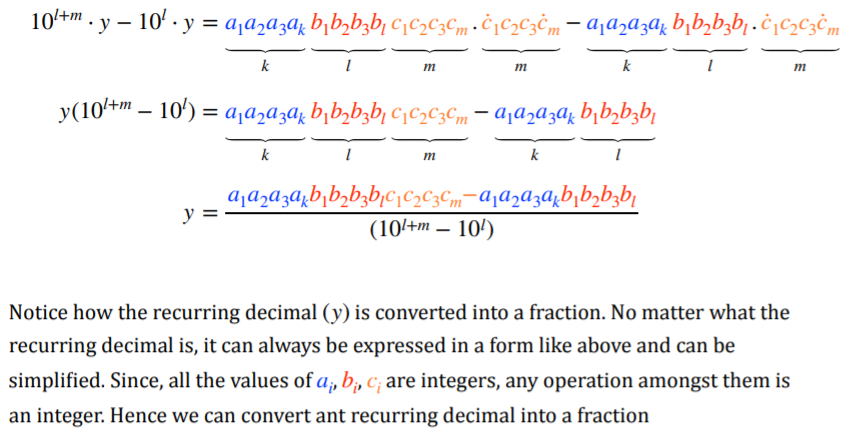ThÃ´ng-sahge from Tiffin School in the UK and Mahdi also used another method to convert the recurring decimals into fractions. ThÃ´ng-sahge also described the process which led to the algebraic generalisation like Mahdi's (above) - linking up the two methods. This is some of ThÃ´ng-sahge's work:
Method 2: Using $S = \dfrac{a}{1-r}$
(This is the formula for the sum $S$ of an infinite geometric series, where the first term is $a$ and the common ratio between each term and the next is $r$)

I know that, for example $0.\dot40\dot5$ is an infinite sum of [the series]:

$0.405 + 0.000405 + 0.000000405 + ...$

Which can be written as:
\begin{align} S &= \tfrac{0.405}{1-0.001}\\ \Rightarrow S &=\tfrac{405}{999}\end{align}

Though I could have stopped here, I wondered whether there was an underlying pattern.

So I tried $1.23\dot4\dot5$

\begin{align} 1.23\dot4\dot5 &=1 + 0.23 + 0.0045 + 0.000045 + ...\\&= 1 + \tfrac{23}{100} + \tfrac{0.0045}{1-0.01}\\&= 1 + \tfrac{23}{100} + \tfrac{45}{9900}\\& = 1 + \tfrac{23\times99+45}{9900}\\& = 1 + \tfrac{23\times(100-1) + 45}{9900}\\&= 1 + \tfrac{2345 - 23}{9900}\end{align}

I tried more examples, and I then noticed, in my example,

\begin{align} 1 + \tfrac{2345 - 23}{9900}&= \tfrac{1(9900) + 2345 - 23}{9900}\\& = \tfrac{12345 - 123}{9900}\end{align}

Wow!!! So if I generalise: $$c_1c_2...c_lb_1b_2...b_m\dot{a_1}a_2...\dot{a_n} = \frac{\left(c_1c_2...c_l.b_1b_2...b_ma_1a_2...a_n\right) - \left(c_1c_2...c_lb_1b_2...b_m\right)}{\underbrace{999...9}_{n\text{ 9s}}\underbrace{000...0}_{m\text{ 0s}}}$$ (for some [positive integers] $l,m,n$)

Which is the same as Mahdi's result.